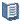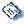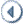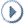Oracle® Fusion Applications Project Management Implementation Guide 11g Release 5 (11.1.5) Part Number E20384-05ContentsContactUsPreviousNext

# 42 Define Project Control Configuration: Manage Spread Curves

This chapter contains the following:

Project and Financial Plan Period Amounts: How They Are Calculated Using Daily Spread Basis

Calculating Distribution Factors for Spread Curves: Examples

Spread curves let you distribute quantity, cost, and revenue amounts automatically across accounting or project accounting periods. You assign a spread curve to each resource class. Planning resources (in the planning resource breakdown structure) inherit the spread curve setting from the associated resource class. You can change the spread curve for the planning resource and for any corresponding task assignments, or budget or forecast lines.

You can create spread curves, use predefined spread curves, or edit them as required. The following is a description of spread curve components (spread points and distribution factors) and predefined spread curves.

Note

When using a daily spread basis, amounts are allocated to each period based on the ratio of the days in the period to the duration of the task assignment.

During financial or project planning, spread points are distributed proportionately across periods. For example, if amounts are to be spread across four periods, Oracle Fusion Projects allocates the combined value of 2.5 spread points to each period. The spread points for each period are the total number of spread points divided by the total number of periods (10 / 4).

Distribution factors are prorated according to the spread points allocated to each period. For example, if \$100 is to be spread across four months for a planning resource that uses an even spread curve (where amounts are distributed evenly), then each period is assigned \$25. The amount assigned to each period is the total amount multiplied by the spread points for the period (2.5 * 10).

Important

Spread points without values are assigned a zero distribution factor and hence corresponding periods are not allocated any amounts.

The following table lists predefined spread curves provided by Oracle Fusion Projects.

Name

Description

Distribution Factors

Even

Ensures a linear distribution of financial or project plan values across periods.

10-10-10-10-10-10-10-10-10-10

Ensures a back-loaded distribution of financial or project plan values across periods. Assigned amounts increase over succeeding periods.

0-5-10-15-20-25-30-35-40-45

Ensures a front-loaded distribution of financial or project plan values across periods. Assigned amounts decrease over succeeding periods.

45-40-35-30-25-20-15-10-5-0

S Curve

Ensures an S-shaped distribution of financial or project plan values across periods.

18-10-8-10-15-17-18-17-15-8

Bell Curve

Ensures a bell-shaped distribution of financial or project plan values across periods. Assignment of plan values is highest in the middle periods.

0-4-10-12-14-12-10-4-0-0

Ensures the spread is based upon the number of days in each financial period throughout the duration of the task assignment. Amounts are proportionally distributed throughout all periods for the duration of the task assignment.

None

## Project and Financial Plan Period Amounts: How They Are Calculated Using Daily Spread Basis

Assign the Daily Spread Basis spread curve to a resource class or planning resource to proportionately distribute budget, forecast, or project plan amounts across periods based on the ratio of the days in each period to the duration of the task assignment.

### Settings That Affect Amount Distribution

You cannot define spread points for the Daily Spread Basis spread curve. Therefore, distribution factors are not calculated. Task assignment start and finish dates determine the number of days in each period, including the first and last periods, and consequently the allocation factor for each period.

Note

Assignment start and finish dates are included in the number of days in the period.

## How Daily Spread Basis Amounts Are Calculated

When calculating period amounts, Oracle Fusion Projects performs the following steps:

1. Determines the number of days in the first and last period within the task assignment duration using assignment start and finish dates.

2. Determines the number of days in the other periods within the assignment duration.

3. Determines the total number of days for the duration of the task assignment.

4. Calculates the allocation factor for each period using the following formula:

Period Allocation Factor = Number of Days in Period / Task Assignment Duration

5. Calculates the periodic amount using the following formula:

Amount = Period Allocation Factor * Total Resource Cost or Revenue

### Example: Standard Accounting Calendar

In this example, a company uses an accounting calendar with periods that are identical to calendar months. A resource is assigned to a task for 121 days, from February 21 until June 21.

The following table shows how the task assignment days are determined, and the resulting allocation factors.

Month

Period Dates

Days in Period

Period Allocation Factor

January

January 1 through January 31

31

0

0

February

February 1 through February 28

28

8

8 / 121 = 0.0661

March

March 1 through March 31

31

31

31 / 121 = 0.2561

April

April 1 through April 30

30

30

30 / 121 = 0.2479

May

May 1 through May 31

31

31

31 / 121 = 0.2561

June

June 1 through June 30

30

21

21 / 121 = 0.1735

121

121 / 121 = 1

### Example: 4-4-5 Accounting Calendar

In this example, a company uses a 4-4-5 accounting calendar, with four weeks in the first and second months of the quarter, and five weeks in the third month of the quarter. A resource is assigned to a task from February 21 until June 21.

The following table shows how the task assignment days are determined, and the resulting allocation factors.

Month

Weeks in Period

Week Number

Ledger Start Date

Ledger End Date

Days in Period

Days in Ledger

Period Allocation Factor

January

4

1

1

7

7

2

8

14

7

3

15

21

7

4

22

28

7

28

0

0

February

4

1

29

4

7

2

5

11

7

3

12

18

7

4

19

25

7

28

5

5 / 121 = 0.0413

March

5

1

26

4

7

2

5

11

7

3

12

18

7

4

19

25

7

5

26

1

7

35

35

35 / 121 = 0.2892

April

4

1

2

8

7

2

9

15

7

3

16

22

7

4

23

29

7

28

28

28 / 121 = 0.2314

May

4

1

30

6

7

2

7

13

7

3

14

20

7

4

21

27

7

28

28

28 / 121 = 0.2314

June

5

1

28

3

7

2

4

10

7

3

11

17

7

4

18

24

7

5

25

1

7

35

25

25 / 121 = 0.2066

121

121/121 = 1

## Calculating Distribution Factors for Spread Curves: Examples

Distribution factors are prorated according to the spread points allocated to each period.

For example, if \$100 is to be spread across four months for a planning resource that uses an even spread curve (where amounts are distributed evenly), then each period is assigned \$25 each. That is, 10 spread points spread over 4 months equals 2.5 spread points per period. Each spread point has a distribution factor of 10.

The following is a description of how distribution factors are calculated for full or partial periods.

### Calculating Weighted Distribution Factors

To continue our previous example: Say our planning resource was using a back-loaded spread curve rather than an even spread curve.

Note

Default distribution factors for a back-loaded spread curve are as follows: 0-5-10-15-20-25-30-35-40-45. Hence the total distribution for the spread curve is 225.

The following table describes how distribution factors are determined and amount allocated over the four planning periods.

Period

Distribution Factor Calculation

Weighted Distribution Factor

Distribution Percentage

Distributed Amount

1

Distribution factors assigned to spread points 1 and 2 plus half of the distribution factor assigned to spread point 3: 0 + 5 + (0.5 * 10)

10.0

4.44%, (10.0/225)

\$4.44

2

Half of distribution factor assigned to spread point 3 plus distribution factors assigned to spread points 4 and 5: (0.5 * 10) + 15 + 20

40.0

17.78%, (40.0/225)

\$17.78

3

Distribution factors assigned to spread points 6 and 7 plus half of the distribution factor assigned to spread point 8: 25 + 30 + (0.5 * 35)

72.5

32.22%, (72.5/225)

\$32.22

4

Half of distribution factor assigned to spread point 8 plus distribution factors assigned to spread points 9 and 10: (0.5 * 35) + 40 + 45

102.5

45.56%, (102.5/225)

\$45.56

Totals

225

100%

\$100

### Deriving Spread Point Values and Prorating Distribution Factors for Partial Periods

Transaction start dates associated with a planning resource or task frequently do not coincide with the start or end dates of a period. In such a case, the actual number of planning resource or task transaction days determines how spread points and constituent distribution factors are allocated to full and partial periods.

Assume that the dates for a task assignment cover only 15 days of the first month (a 30-day month) of a four-month planning period. That first month represents the value of 0.5 spread points.

In such a case, the spread point value for each full period is calculated by dividing the total number of spread points (10) by the number of periods corresponding to the transaction (3.5). In other words, spread point values are as follows:

• Full period : 10/3.5 = 2.8571

• Partial Period: (10/3.5) * 0.5 = 1.4287